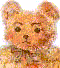BillHung.Net

CS 61A Labs

Lab 1 - pigl.scm

(define (pigl wd)
(if (pl-done? wd)
(word wd 'ay)
(pigl (word (bf wd) (first wd)))))

(define (pl-done? wd)
(vowel? (first wd)))

(define (vowel? letter)
(member? letter '(a e i o u)))

(transcript-on "lab1a") ; This starts a transcript file with the name lab1a.
(pigl ’scheme) ; Try out your program.
; Feel free to try more test cases here!
(trace pigl) ; This is a debugging aid. Watch what happens
(pigl ’scheme) ; when you run a traced procedure.
(transcript-off)
(exit)

lab1a trace

okay
okay
STk> (pigl 'scheme)
emeschay
STk> (trace pigl)
okay
STk> (pigl 'scheme)
.. -> pigl with wd = scheme
.... -> pigl with wd = chemes
...... -> pigl with wd = hemesc
........ -> pigl with wd = emesch
........ <- pigl returns emeschay
...... <- pigl returns emeschay
.... <- pigl returns emeschay
.. <- pigl returns emeschay
emeschay
STk>

Lab1 - plural.scm

(define (plural wd)
(if (equal? (last wd) 'y)
(word (bl wd) 'ies)
(word wd 's)))

Square Root in Scheme [SICP 1.1.7]

(define (sqrt x)
(sqrt-iter 1.0 x))
(define (sqrt-iter guess x)
(if (good-enough? guess x)
guess
(sqrt-iter (improve guess x) x)))
(define (good-enough? guess x)
(< (abs (- (square guess) x)) 0.001))
(define (improve guess x)
(average guess (/ x guess)))

Newton's Successive Approximations for finding the root of a number

 Guess Quotient Average 1 (2/1) = 2 ((2 + 1)/2) = 1.5 1.5 (2/1.5) = 1.3333 ((1.3333 + 1.5)/2) = 1.4167 1.4167 (2/1.4167) = 1.4118 ((1.4167 + 1.4118)/2) = 1.4142 1.4142 ... ...

;Lab 1 Last Letter ies

(define (plural wd)
(if (equal? (last wd) 'y)
(if (vowel? (last (bl wd)))
(word wd 's)
(word (bl wd) 'ies))
(word wd 's)))

;Lab 1 answer=a^2 + b^2 where a, b are the bigger of a, b, c

(define (sum-square-large a b c)
(define (square x) (* x x))
(define (sumsq x y) (+ (square x) (square y)))
(if (>= a b)
(if (>= b c)
(sumsq a b)
(sumsq a c))
(if (>= a c)
(sumsq a b)
(sumsq b c))))

;Lab 1 Remove Duplicated words

(define (dupls-removed sent)
(cond ((empty? sent) '())
((member? (first sent) (bf sent))
(dupls-removed (bf sent)))
(else (sentence (first sent) (dupls-removed (bf sent))))))

> (dupls-removed ’(a b c a e d e b))

(c a d e b)

> (dupls-removed ’(a b c))

(a b c)

> (dupls-removed ’(a a a a b a a))

(b a)

3

Lab 2A Question #5
(define (type-check proc pred wd)
(if ( pred wd
)
(proc wd)
#f ;false
);if
);define

Lab2A #6
####################
(define (make-safe proc pred?)
lambda (wd)
((if ( pred? wd
)
(proc wd)
#f ;false
);if
);lambda
) ;define

Reference

SICP = Structure and Interpretation of Computer Programs. Second Edition. Harnold Abelson, Gerald Jay Sussman, and Julie Susman. ISBN 0262011530. 657 pages. The MIT Press. July 1996. Full Text Online http://mitpress.mit.edu/sicp/full-text/book/book.html